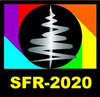#Synchrotron and Free electron laser Radiation: generation and application (SFR-2020)

13-17 July 2020
Zoom
Asia/Novosibirsk timezone

## Enhancing resolution of terahertz surface plasmon resonance microscopy by ghost imaging using FEL radiation

14 Jul 2020, 18:40
20m
Zoom 860 5034 1820 (Zoom)

### Speaker

Ildus Khasanov (Scientific and Technological Center of Unique Instrumentation of the Russian Academy of Sciences)

### Description

Surface plasmon resonance (SPR) microscopy is one of the most sensitive label-free microscopy method, however, it has a low lateral resolution. This drawback is primarily caused by the fact that surface plasmon polaritons (SPPs) excited by terahertz (THz) radiation propagate from their excitation spot to macro distances (about ~100 λ), thereby blurring the observed region by analogy with a scattering medium. To eliminate this disadvantage, we propose to adapt method known as ghost imaging (GI), which is notable for its tolerance to environmental aberrations between object and camera.
The GI established itself in the classical optical visible-range nanoscopy, allowing to obtain a high resolution. In the report we will present an optical scheme of SPR microscopy for the THz range with an additional optical arm to implement the classical GI. In the GI the image of an object $O(x,y)$ is reconstructed by calculating the second-order correlation function (mutual intensity) between the spatial distribution of the intensity $P(x,y)$ of the probing beam (patterns) and the integral intensity $S$ (registered by a single-pixel receiver) of the reflected (or transmitted) light in two optical arms $(x,y)$ and ($x'$,$y'$):
$$O(x,y) \propto \sum_{i=1}^N (S_i- \langle S \rangle)({P(x,y)}_i-\langle P(x,y) \rangle)\rangle = \langle {P(x,y)}_i \cdot S_i \rangle - \langle P(x,y) \rangle \cdot \langle S \rangle,$$ where $\langle \dots \rangle = \frac{1}{N} \sum_{i=1}^N$ is the averaging operator, N is the number of independent patterns, $R(x',y')$ is the object response function, $S = \int R(x',y') {P(x',y')}_i dx' dy'$. The restored image is called a ghost image, because it uses information about the spatial structure of light that did not interact with the object.
In the THz range, the classical GI in the far field has not yet been demonstrated because it requires a THz radiation source having a sufficient second-order coherence length exceeding the wavelength by at least 2-3 orders of magnitude. This requirement is met by light from a powerful coherent THz source of radiation transmitted through a transparent turbulent medium or reflected from a rough surface, as a result of which the radiation acquires the statistical properties of a thermal source. To implement the classical GI in the THz range, we propose to use the Novosibirsk free electron laser (NovoFEL) as a THz radiation source. The high beam power of the NovoFEL provides a speckle structure with a pronounced profile, which is necessary to achieve a high level of contrast in the obtained image. And large coherence length of radiation of the NovoFEL is necessary to ensure a large degree of correlation between the beams emerging from the beamsplitter, which allows reconstructing an image with a lower noise level.
The work was supported by the Russian Foundation for Basic Research (project 20-52-54004).

### Summary

This report will consider the advantages of implementation the ghost imaging method to surface plasmon-polariton resonance microscopy in the THz range and presents an analysis of factors affecting the resolution of proposed method.

### Primary authors

Ildus Khasanov (Scientific and Technological Center of Unique Instrumentation of the Russian Academy of Sciences) Vasily Gerasimov (Budker Institute of Nuclear Physics SB RAS) Prof. Alexey Nikitin Prof. Boris Knyazev Lidia Zykova (Scientific and Technological Center of Unique Instrumentation of the Russian Academy of Sciences)